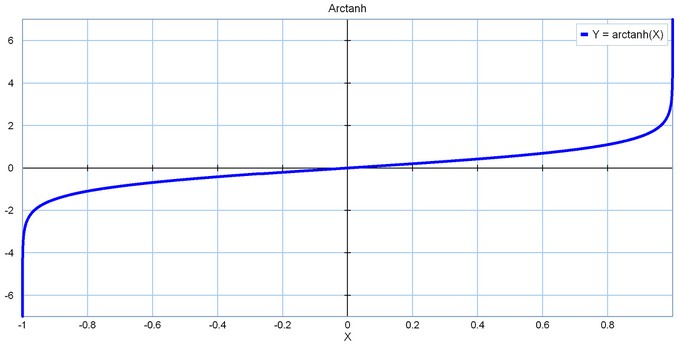﻿ 20-sim webhelp > Language Reference > Functions > Trigonometric > arctanh

# arctanh

Y = arctanh(X);

## Description

Returns the hyperbolic arctangent of the elements of X.

## Examples

b = sin(time);

a = arctan(b);

X = [sin(time);-0.5*cos(time)];

Y = arctanh(X);

## Limitations

Y and X must have the same size. The elements of X must be in the range [-1 ,1].